Question

# The effectiveness of a blood-pressure drug is being investigated. An experimenter finds that, on average, the...

The effectiveness of a blood-pressure drug is being investigated. An experimenter finds that, on average, the reduction in systolic blood pressure is 70.2 for a sample of size 25 and standard deviation 17.7. Estimate how much the drug will lower a typical patient's systolic blood pressure (using a 98% confidence level). Assume the data is from a normally distributed population.

Enter your answer as a tri-linear inequality accurate to three decimal places.

__________< μ < ______

Solution :

Given that,

sample size = n = 25

Degrees of freedom = df = n - 1 = 25 - 1 = 24

t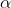/2,df = 2.492

Margin of error = E = t/2,df * (s /n)

= 2.492 * (17.7 /25)

Margin of error = E = 8.822

The 98% confidence interval estimate of the population mean is,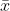- E <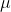<+ E

70.2 - 8.822 << 70.2 + 8.822

61.378 << 79.022

#### Earn Coins

Coins can be redeemed for fabulous gifts.

Similar Homework Help Questions
• ### The effectiveness of a blood-pressure drug is being investigated. An experimenter finds that, on average, the reduction...

The effectiveness of a blood-pressure drug is being investigated. An experimenter finds that, on average, the reduction in systolic blood pressure is 32.6 for a sample of size 20 and a standard deviation of 8.8. Estimate how much the drug will lower a typical patient's systolic blood pressure (using a 98% confidence level). Assume the data is from a normally distributed population. Enter your answer as a tri-linear inequality accurate to three decimal places. < μ <

• ### The effectiveness of a blood-pressure drug is being investigated. An experimenter finds that, on average, the...

The effectiveness of a blood-pressure drug is being investigated. An experimenter finds that, on average, the reduction in systolic blood pressure is 68.8 for a sample of size 27 and standard deviation 7.4. Estimate how much the drug will lower a typical patient's systolic blood pressure (using a 99% confidence level). Assume the data is from a normally distributed population. Enter your answer as a tri-linear inequality accurate to three decimal places. ____ < μ < ____

• ### The effectiveness of a blood-pressure drug is being investigated. An experimenter finds that, on average, the...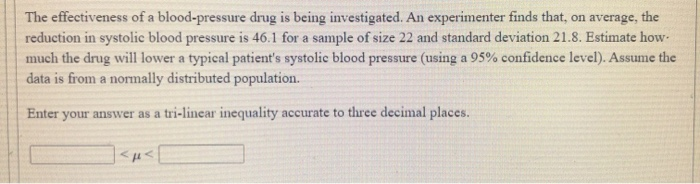The effectiveness of a blood-pressure drug is being investigated. An experimenter finds that, on average, the reduction in systolic blood pressure is 46.1 for a sample of size 22 and standard deviation 21.8. Estimate how much the drug will lower a typical patient's systolic blood pressure (using a 95% confidence level). Assume the data is from a normally distributed population. Enter your answer as a tri-linear inequality accurate to three decimal places. <μ<

• ### The effectiveness of a blood pressure drug is being investigated. An experimenter finds that, on average,...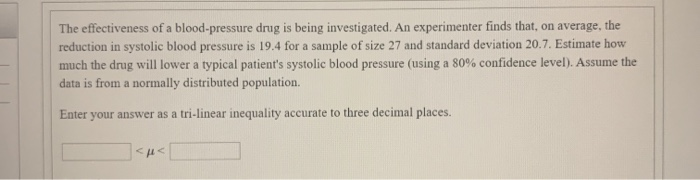The effectiveness of a blood pressure drug is being investigated. An experimenter finds that, on average, the reduction in systolic blood pressure is 19.4 for a sample of size 27 and standard deviation 20.7. Estimate how much the drug will lower a typical patient's systolic blood pressure (using a 80% confidence level). Assume the data is from a normally distributed population. Enter your answer as a tri-linear inequality accurate to three decimal places.

• ### The effectiveness of a blood-pressure drug is being investigated. An experimenter finds that, on average, the...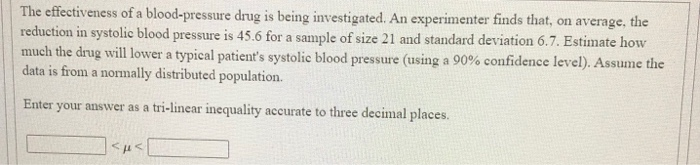The effectiveness of a blood-pressure drug is being investigated. An experimenter finds that, on average, the reduction in systolic blood pressure is 45.6 for a sample of size 21 and standard deviation 6.7. Estimate how much the drug will lower a typical patient's systolic blood pressure (using a 90% confidence level). Assume the data is from a normally distributed population Enter your answer as a tri-linear inequality accurate to three decimal places. <<

• ### The effectiveness of a blood-pressure drug is being investigated. An experimenter finds that, on average, the...

The effectiveness of a blood-pressure drug is being investigated. An experimenter finds that, on average, the reduction in systolic blood pressure is 71.4 for a sample of size 28 and standard deviation 20.4. Estimate how much the drug will lower a typical patient's systolic blood pressure (using a 95% confidence level). Assume the data is from a normally distributed population. Enter your answer as a tri-linear inequality accurate to three decimal places. < μμ <

• ### The effectiveness of a blood-pressure drug is being investigated. An experimenter finds that, on average, the...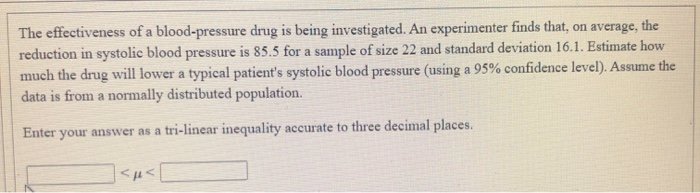The effectiveness of a blood-pressure drug is being investigated. An experimenter finds that, on average, the reduction in systolic blood pressure is 85.5 for a sample of size 22 and standard deviation 16.1. Estimate how much the drug will lower a typical patient's systolic blood pressure (using a 95% confidence level). Assume the data is from a normally distributed population. Enter your answer as a tri-linear inequality accurate to three decimal places. μς

• ### The effectiveness of a blood-pressure drug is being investigated. An experimenter finds that, on average, the...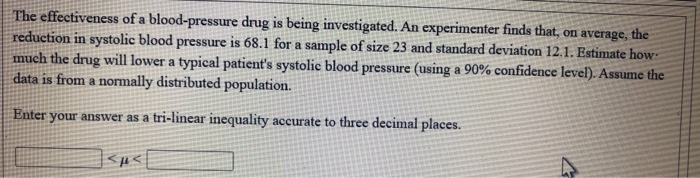The effectiveness of a blood-pressure drug is being investigated. An experimenter finds that, on average, the reduction in systolic blood pressure is 68.1 for a sample of size 23 and standard deviation 12.1. Estimate how much the drug will lower a typical patient's systolic blood pressure (using a 90% confidence level). Assume the data is from a normally distributed population. Enter your answer as a tri-linear inequality accurate to three decimal places. <<

• ### The effectiveness of a blood-pressure drug is being investigated. An experimenter finds that, on average, the...

The effectiveness of a blood-pressure drug is being investigated. An experimenter finds that, on average, the reduction in systolic blood pressure is 42.7 for a sample of size 519 and standard deviation 19.3. Estimate how much the drug will lower a typical patient's systolic blood pressure (using a 95% confidence level). Enter your answer as a tri-linear inequality accurate to one decimal place (because the sample statistics are reported accurate to one decimal place). < μ<

• ### The effectiveness of a blood-pressure drug is being investigated. An experimenter finds that, on average, the...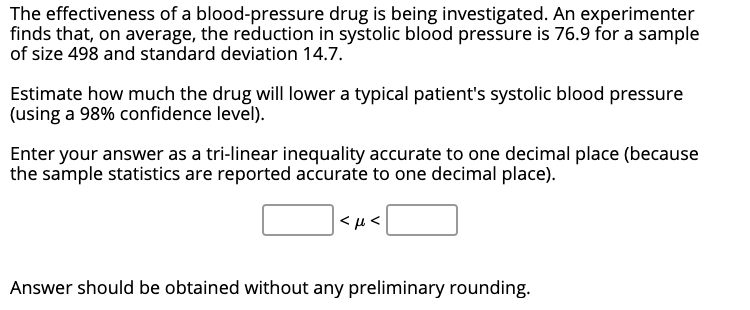The effectiveness of a blood-pressure drug is being investigated. An experimenter finds that, on average, the reduction in systolic blood pressure is 76.9 for a sample of size 498 and standard deviation 14.7. Estimate how much the drug will lower a typical patient's systolic blood pressure (using a 98% confidence level). Enter your answer as a tri-linear inequality accurate to one decimal place (because the sample statistics are reported accurate to one decimal place). <u Answer should be obtained without...